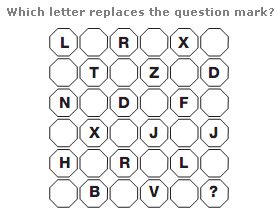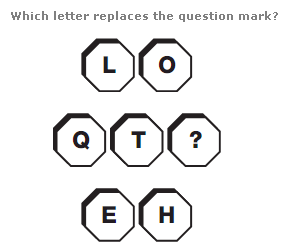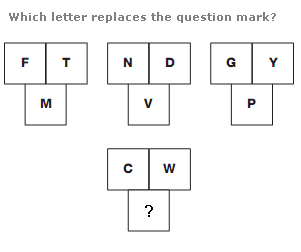# Puzzles - Missing letters puzzles

### Exercise :: Missing letters puzzlesAnswer : P Explanation : Starting in the top left corner and moving clockwise in a spiral towards the centre, letters follow the alphabetic sequence, skipping 5 letters at a time, written in alternate squares of the diagram.Answer : C Explanation : The sum of the numerical values of pairs of letters, opposite each other in the diagram, is always 20 (the numerical value of the central letter).Answer : M Explanation : In each diagram, start with the upper left box, and move anti-clockwise around the others. Letters advance through the alphabet in steps of 7 for the upper left diagram, 8 for the middle, then 9, then 10.